Resultant Force

As the name suggests, this is the force that is the result of two, or more, forces acting on an object. As forces are vector quantities, the forces cannot just be added by only taking into account the magnitude (size). The directions of the forces have also to be considered. If the forces are all in a straight line, the addition (or subtraction) of the forces are straightforward, which does not have to involve angles.

However, when the
forces are not in a straight line, methods such as the triangle / parallelogram of forces and/or trigonometry can be used to determine the resultant force. In the example below, trigonometry is employed to help solve the resultant force of A and B.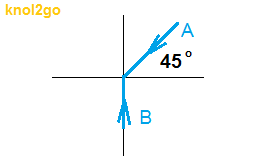Resolving force A into component forces in the vertical and horizontal directions: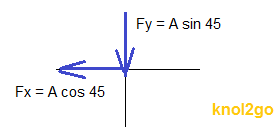Next, only the forces and/or component forces in the vertical and horizontal directions are dealt with.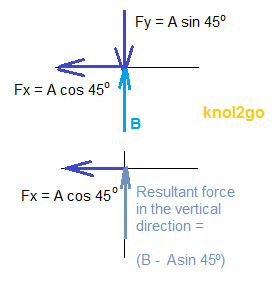Using the Pythagorean theorem, the magnitude of the resultant force can be calculated, and using trigonometry, the angle or direction (angle) can be determined.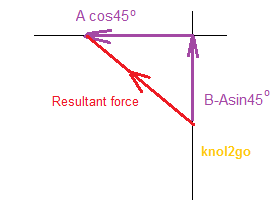If this knol is helpful, please Like and Share it. Thanks.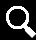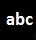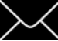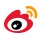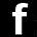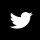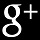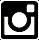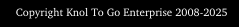Contoh-contoh melibatkan daya paduan adalah seperti yang tertera di atas.

Sekira knol ini memanfaatkan anda, sila tekan Like dan Kongsikannya. Terima kasih.